# Spatial Analytics

Geography, some economics and data analysis.

# Plotting New Zealand with R

### Phil Donovan / 2018-11-01

Presently, there is a handy series of blogs that have just been released demonstrating ggplot2’s new integration with the simple features package by Mel Moreno and Mathieu Basille. I just wanted to provide a quick demo in New Zealand. This code uses my R package which contains the New Zealand census data called nzcensr.

First, I’ll just load the libraries and get the New Zealand regional data.

library(sf)
library(ggspatial)
library(tidyverse)
library(ggthemes)
library(nzcensr)
library(ggrepel)

# Regions data is lazy loaded by the nzcensr and simplify
regions_simple_1000 <- st_simplify(regions, dTolerance = 1000)

# Set the theme
theme_set(theme_tufte())

We can make a simple plot of this by

ggplot() +

geom_sf(data = regions_simple_1000) +

ggtitle("The Regions of New Zealand",
subtitle = "From the 2013 NZ Census") +

# Add north arrow and scale bar
annotation_north_arrow(location = "br", which_north = "true",
style = north_arrow_fancy_orienteering) +
annotation_scale(location = "br", width_hint = 0.5) +

# Tinker with the theme a bit.
theme(panel.grid.major = element_line(color = gray(.5),
linetype = "dashed", size = 0.5),
panel.background = element_rect(fill = "white"))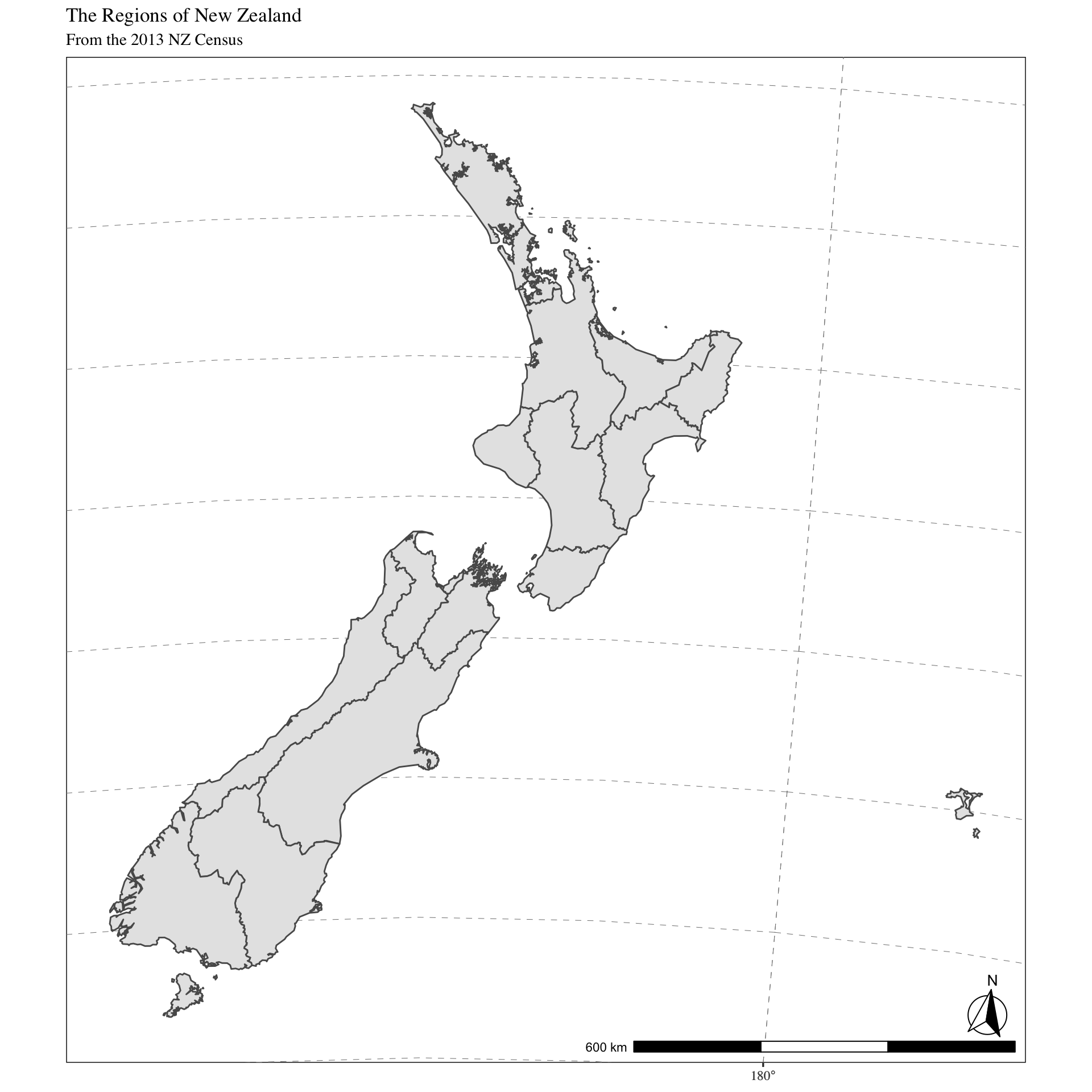This is fairly nifty, and obviously the integration of ggplot with sf means that you can get a lot of the features of ggplot2 with spatial data; such as faceting.

However, say we wanted to show the territorial authorities for each region. We can do this by just adding an additional geom_sf with the data specified.

# Simplify TA data
tas_simple_1000 <- st_simplify(tas, dTolerance = 1000)

ggplot(regions_simple_1000) +

geom_sf() +
geom_sf(data = tas_simple_1000, fill = NA, linetype = "dotted") +

ggtitle("The Regions and Territorial Authorities of New Zealand",
subtitle = "From the 2013 NZ Census") +

# Add north arrow and scale bar
annotation_north_arrow(location = "br", which_north = "true",
style = north_arrow_fancy_orienteering) +
annotation_scale(location = "br", width_hint = 0.5) +

# Tinker with the theme a bit.
theme(panel.grid.major = element_line(color = gray(.5),
linetype = "dashed", size = 0.5),
panel.background = element_rect(fill = "white"))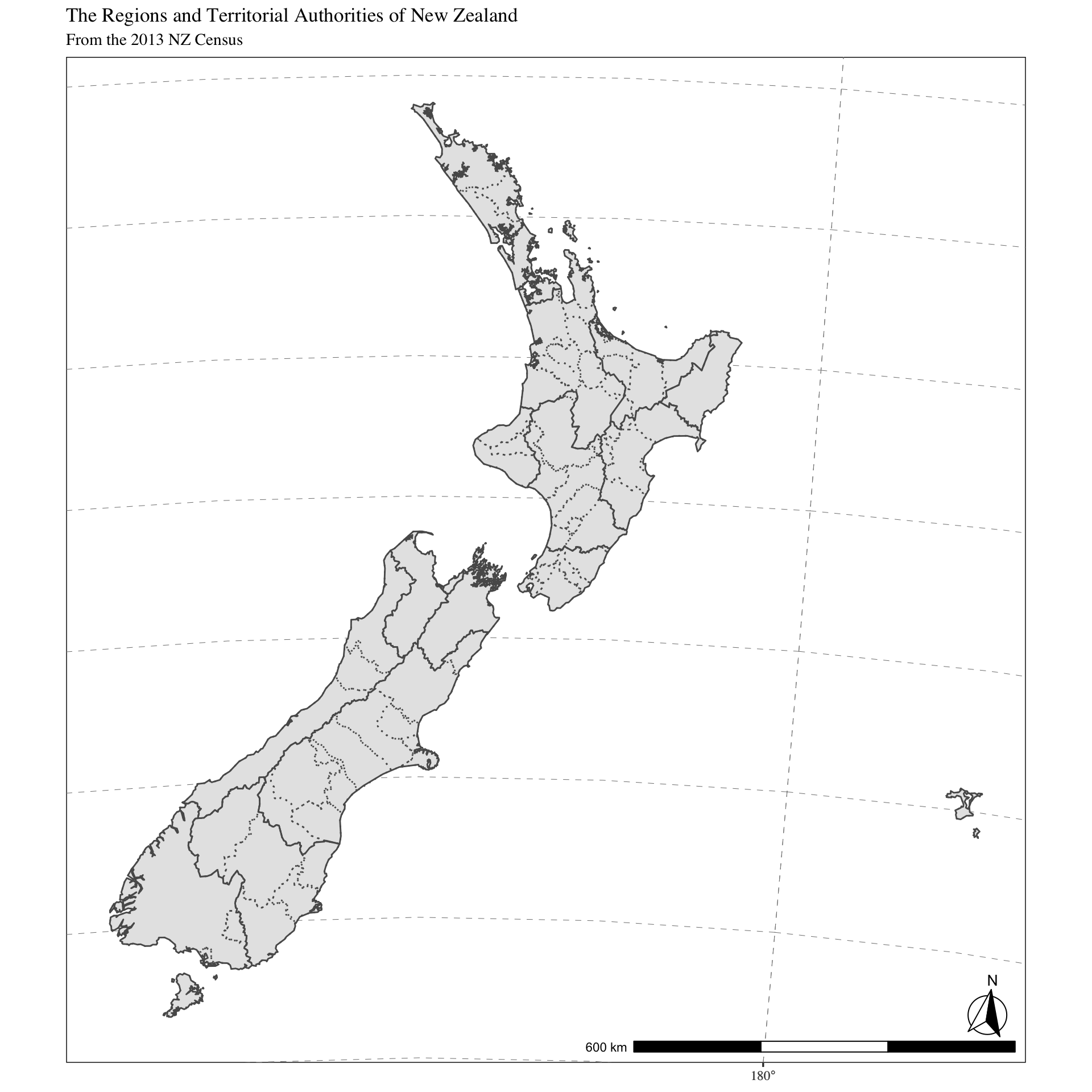Cool, but which TAs are which? Perhaps we should add some text labels.

# Unfortunately ggrepel cannot take sf objects so we convert
# the tas to centroids and then add the x, y to the ta data
tas_centroids <- st_centroid(tas) %>%
bind_cols(st_coordinates(.) %>% data.frame)

# Let's also strip away district from label names as they
# just clutter the presentation a little.
tas_centroids <-
mutate(tas_centroids,
TA2013_NAM = str_replace(TA2013_NAM, " District", ""))

ggplot(regions_simple_1000) +

geom_sf() +
geom_sf(data = tas_simple_1000, fill = NA, linetype = "dotted") +
geom_text_repel(data = tas_centroids,
aes(x = X, y = Y, label = TA2013_NAM),
alpha = 0.75,
fontface = "italic", family = "serif",
nudge_x = c(1, -1.5, 2, 2, -1),
nudge_y = c(0.25, -0.25, 0.5, 0.5, -0.5)) +

ggtitle("The Regions and Territorial Authorities of New Zealand",
subtitle = "From the 2013 NZ Census") +

# Add north arrow and scale bar
annotation_north_arrow(location = "br", which_north = "true",
style = north_arrow_fancy_orienteering) +
annotation_scale(location = "br", width_hint = 0.5) +

# Tinker with the theme a bit.
theme_tufte() +
theme(panel.grid.major = element_line(color = gray(.5),
linetype = "dashed", size = 0.5),
panel.background = element_rect(fill = "white"),
axis.title = element_blank())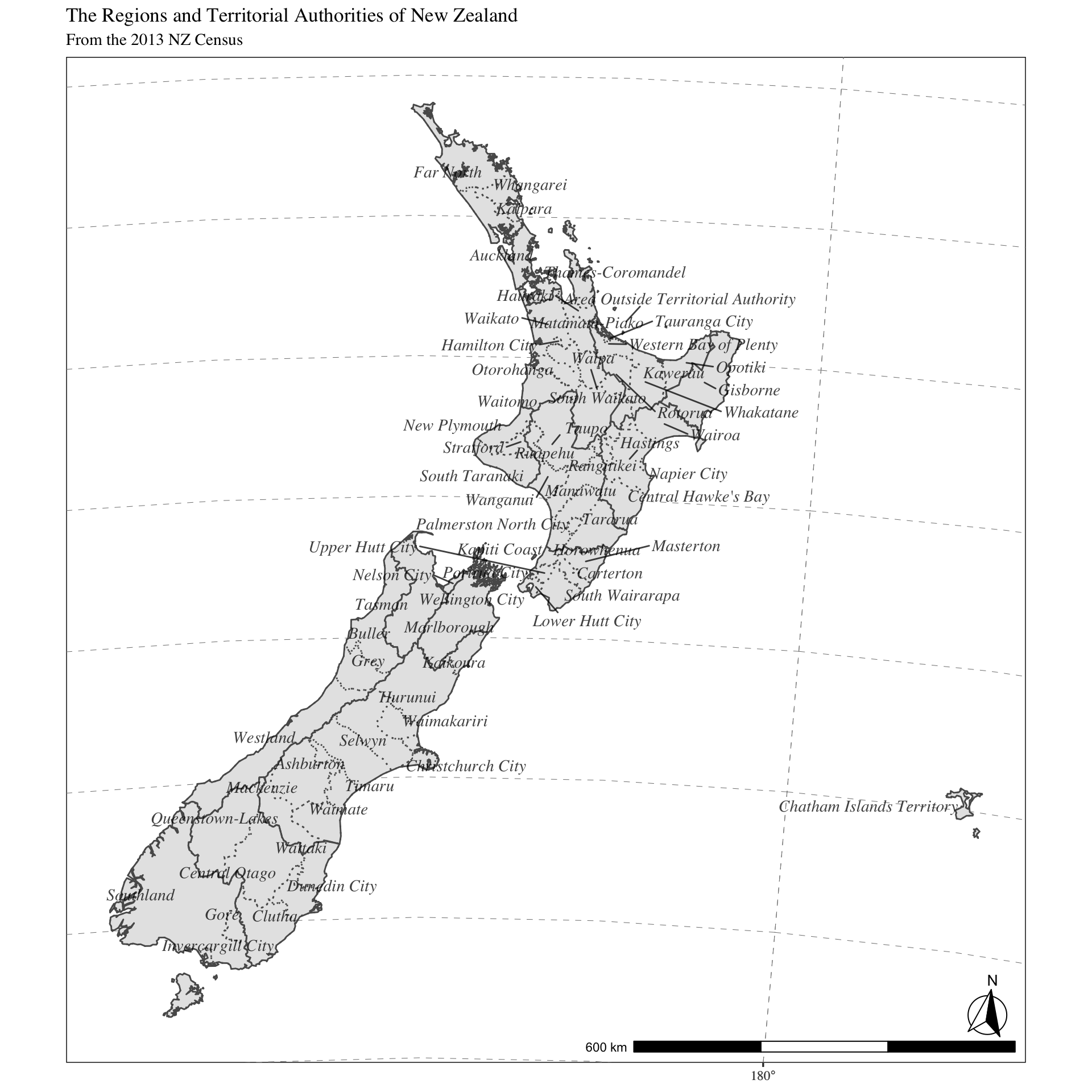It’s a busy plot, but quite cool I think. But it would be quite cool to rearrange things to make the utilisation of space better. I’m thinking that it would be cool to create the map with an inset of the Chatham Islands so that it was less expansive; much like you see the Alaska being represented in maps of the USA. So let’s do it!

# We'll use the cowplot library to easily inset ggplot graphs / maps.
library(cowplot)

# Split the data between Chatham Islands and the rest of NZ.
chathams <- filter(regions_simple_1000, REGC2013 == 99)
chatham_tas <- filter(tas_simple_1000, str_detect(TA2013_NAM, "Chatham"))
chatham_tas_point <- filter(tas_centroids, str_detect(TA2013_NAM, "Chatham"))

nz_regions <- filter(regions_simple_1000, REGC2013 != 99)

nz_tas <- filter(tas_simple_1000,
!str_detect(TA2013_NAM, "Chatham|Area Outside"))

nz_tas_point <- filter(tas_centroids,
!str_detect(TA2013_NAM, "Chatham|Area Outside"))

# Make plots
# First plot is jsut the plot of the mainland excluding the Chathams.
nz_plot <-

# Create ggplot object
ggplot(nz_regions) +

geom_sf() +
geom_sf(data = nz_tas, fill = NA, linetype = "dotted") +
geom_text_repel(data = nz_tas_point,
aes(x = X, y = Y, label = TA2013_NAM),
alpha = 0.75,
fontface = "italic", family = "serif",
nudge_x = c(1, -1.5, 2, 2, -1),
nudge_y = c(0.25, -0.25, 0.5, 0.5, -0.5)) +

ggtitle("The Regions and Territorial Authorities of New Zealand",
subtitle = "From the 2013 NZ Census") +

# Add north arrow and scale bar
annotation_north_arrow(location = "bl", which_north = "true",
style = north_arrow_fancy_orienteering) +
annotation_scale(location = "bl", width_hint = 0.5) +

# Tinker with the theme a bit.
theme_tufte() +
theme(panel.grid.major = element_line(color = gray(.5),
linetype = "dashed", size = 0.5),
panel.background = element_rect(fill = "white"),
axis.title = element_blank())

# Chathams
chatham_plot <-

# Create ggplot object
ggplot(chathams) +

geom_sf() +
geom_sf(data = chatham_tas, fill = NA, linetype = "dotted") +
geom_text_repel(data = chatham_tas_point,
aes(x = X, y = Y, label = TA2013_NAM),
alpha = 0.75,
fontface = "italic", family = "serif") +

# Tinker with the theme a bit.
theme_bw() +
theme(panel.grid.major = element_line(color = "white"),
panel.grid.minor = element_blank(),
panel.background = element_rect(fill = "white"),
axis.title = element_blank(),
axis.ticks = element_blank(),
axis.text = element_blank(),
axis.line = element_blank())

ggdraw(nz_plot) +
draw_plot(chatham_plot, width = 0.16, height = 0.16, x = 0.65, y = 0.05)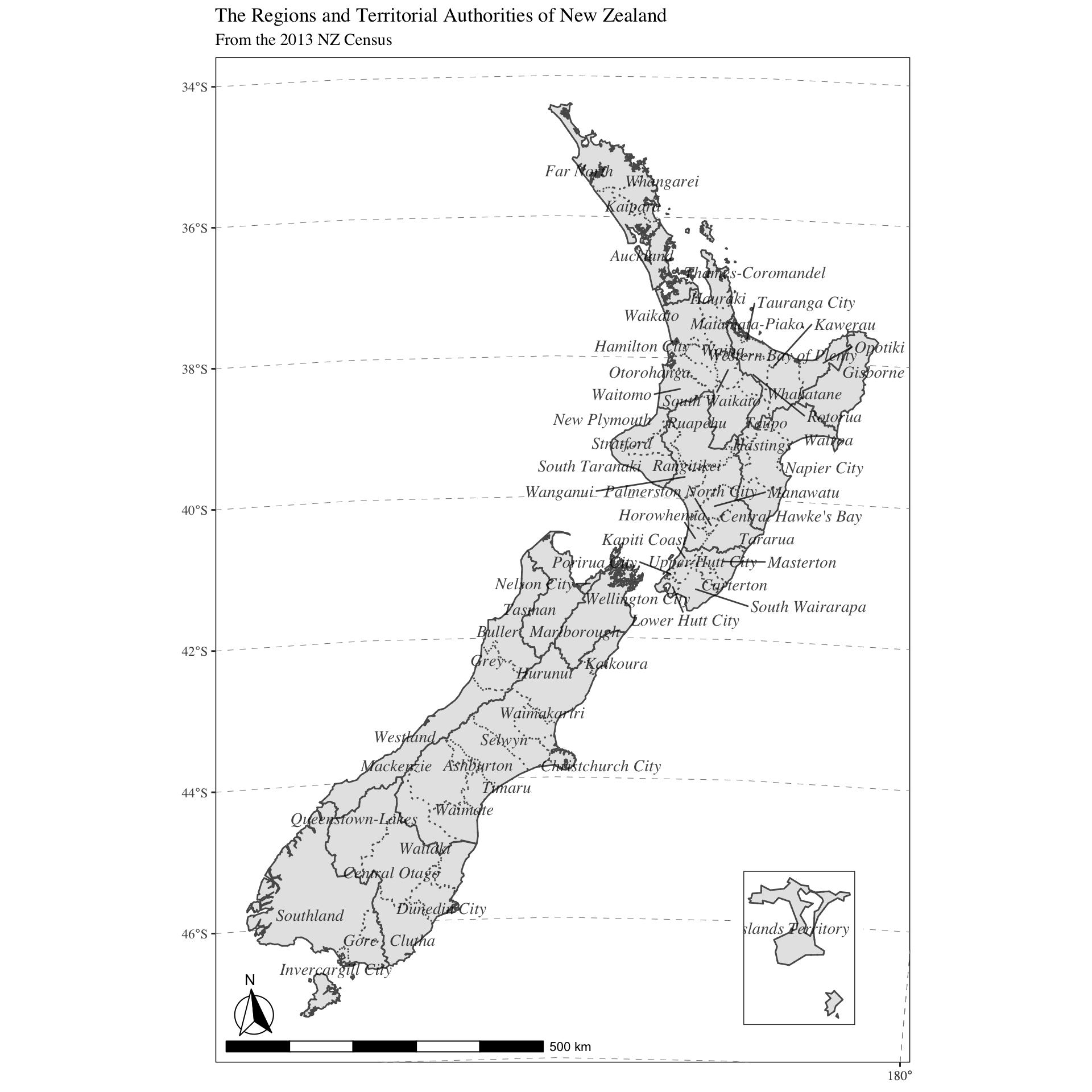That does tighten the map up somewhat and although it is still quite cluttered, it does look rather nifty in my opinion.

As a final demonstration, I should probably add some colour! I think it would be suitable to do this for the regions, to demonstrate which region is which. This only requires an additional parameter being added to the geom_sf(aes(fill = REGC2013_N)):

nz_plot <-

# Create ggplot object
ggplot(nz_regions) +

geom_sf(aes(fill = REGC2013_N)) +
geom_sf(data = nz_tas, fill = NA, linetype = "dotted") +
geom_text_repel(data = nz_tas_point,
aes(x = X, y = Y, label = TA2013_NAM),
alpha = 0.75,
fontface = "italic", family = "serif",
nudge_x = c(1, -1.5, 2, 2, -1),
nudge_y = c(0.25, -0.25, 0.5, 0.5, -0.5)) +

ggtitle("The Regions and Territorial Authorities of New Zealand",
subtitle = "From the 2013 NZ Census") +
labs(fill = "Region") +

# Add north arrow and scale bar
annotation_north_arrow(location = "bl", which_north = "true",
style = north_arrow_fancy_orienteering) +
annotation_scale(location = "bl", width_hint = 0.5) +

# Tinker with the theme a bit.
theme_tufte() +
theme(panel.grid.major = element_line(color = gray(.5),
linetype = "dashed", size = 0.5),
panel.background = element_rect(fill = "white"),
axis.title = element_blank())

nz_plot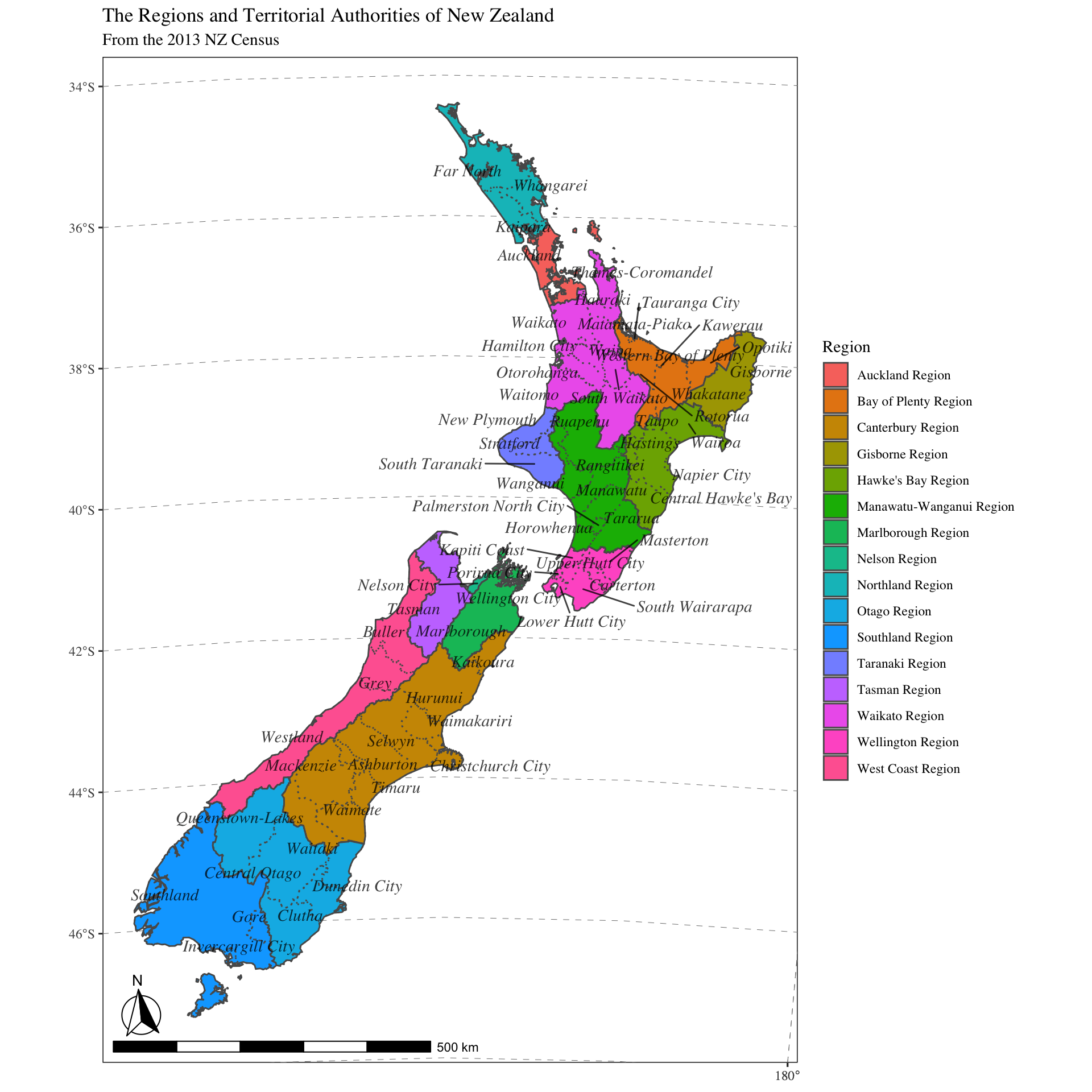I guess one question remains, so what? Maps like this are easily made in other software programs such as ArcGIS, QGIS, MapInfo etc! Well, there would be several reasons to make maps in R: (1) it is scripted, so it can easily be changed and quickly updated; (2) R encompasses a much bigger ecosystem of statistical analysis e.g. it can do more than GIS systems; (3) building on the previous, it means you can stay with the R environment for map making. Furthermore, this is only the beginning of sf integration and I am certain that it will improve and get even more powerful.